# What is Repeated Subtraction?

Repeated subtraction is a useful way to introduce students to division. By using this method to solve division problems, students gain a deeper understanding of how to divide numbers into equal groups.Author
Katie WickliffPublished
October 11, 2023# What is Repeated Subtraction?

By using repeated subtraction to solve division problems, students gain a deeper understanding of how to divide numbers into equal groups.Author
Katie WickliffPublished
Oct 11, 2023# What is Repeated Subtraction?

Repeated subtraction is a useful way to introduce students to division. By using this method to solve division problems, students gain a deeper understanding of how to divide numbers into equal groups.Author
Katie WickliffPublished
October 11, 2023Key takeaways

• The repeated subtraction method helps students understand division as creating equal groups.
• The repeated subtraction method can help students learn division because it asks them to perform a skill (subtraction) that they have already learned.
• Repeated subtraction is one of several effective division strategies.

When your child is learning division, it helps to have several strategies to strengthen their understanding and boost their confidence. One effective introductory strategy is repeated subtraction. The repeated subtraction method provides students with a concrete way to see the relationship between division and subtraction.

This article outlines how to perform repeated subtraction to solve simple division problems. We also provide several examples, practice problems, and additional practice resources. Let’s get started!

## What is Repeated Subtraction?Repeated subtraction is a way to solve certain division problems in math.  This method takes away the same number from a larger number until you reach zero— or a number smaller than the one being subtracted. The number of times you “jump back” is the answer to the division problem.

## Division by Repeated SubtractionUsing the repeated subtraction method is helpful when introducing students to division because it allows them to use a familiar math skill to help solve a new type of problem. Students feel like they are building on their knowledge, rather than learning something completely unfamiliar.

Using the repeated subtraction process as a foundation can help students gain the confidence to tackle more complex division problems with varying strategies, such as short or long division.

## Repeated Subtraction ExamplesLet’s look at a few examples of division as repeated subtraction.

### Example 1

16÷4= ?

To divide a number using repeated subtraction, start with the number you are dividing. This number is also called the dividend. In this example, that number is 16.

Then, subtract the number you are dividing by. This number is also called the divisor. In this example, that number is 4.

16-4= 12

There are more than 4 left, so we subtract again.

12-4=8

We still have more than 4 left, so we’ll subtract until we get to zero or a number smaller than four:

8-4=4

One last time:

4-4= 0

Now that we’ve reached zero, we count the number of times we subtracted 4 from 16. We subtracted 4 times, so our answer (or quotient) is 4.

16÷4=4

### Example 2

Piper has 24 stickers. She gives an equal amount of stickers to 6 friends. How many stickers does each friend get?

To solve this division problem using repeated subtraction, we first look at the dividend. In this example, that number is 24.

Then, we repeatedly subtract the divisor until we reach zero or a number smaller than the divisor. In this example, that number is 6.

24-6=18

18-6=12

12-6=6

6-6=0

Now that we reached zero, we count the number of times we subtracted the divisor (6) from the dividend (24).

We subtracted the divisor 4 times.

24 ÷ 6= 4

Piper gave 6 friends 4 stickers apiece.

## Repeated Subtraction Practice ProblemsSolve each of these problems using the repeated subtraction method. Be sure to show your work! When you’ve worked through all of these, find more helpful practice problems with DoodleLearning’s interactive math app.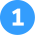John has 8 crayons. If he shares them equally with 4 friends, how many crayons does each friend get?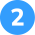Solve 20÷ 4 using repeated subtraction.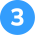Stella brought 16 oranges to the soccer game. If there are 8 players on the team, how many oranges does each player get?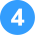Twenty-one students are participating in the school relay race. If each relay team can have 3 members, how many relay teams will there be altogether?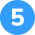If 36 is the dividend, and 9 is the divisor, what is the quotient?8-4=4

4-4=0

8÷ 4=2

John gave each friend 2 crayons.20÷ 4=?

20-4=16

16-4=12

12-4=8

8-4=4

4-4=0

20 ÷ 4 = 516÷ 8=?

16-8=8

8-8=0

16÷ 8=2

Each soccer player gets 2 oranges.21÷ 3=?

21-3=18

18-3=15

15-3=12

12-3=9

9-3=6

6-3=3

3-3=0

21÷ 3=7

There will be 7 relay teams altogether.36÷ 9=?

36-9=27

27-9=18

18-9=9

9-9=0

36÷ 9=4

The quotient is 4.Repeated subtraction is a method students can use to solve some division problems. This method asks students to repeatedly subtract the divisor from the dividend until they reach zero or a lesser number.

Yes, division is repeated subtraction. Using repeated subtraction for division problems allows students to visualize how to break numbers down into equal groups.

An example of repeated subtraction is solving 12÷ 3 this way:

12-3=9

9-3=6

6-3=3

3-3=0

In this repeated subtraction example, 3 is subtracted 4 times, so the answer to 12÷ 3 is 4.Lesson credits

Katie Wickliff

Katie holds a master’s degree in Education from the University of Colorado and a bachelor’s degree in both Journalism and English from The University of Iowa. She has over 15 years of education experience as a K-12 classroom teacher and Orton-Gillingham certified tutor. Most importantly, Katie is the mother of two elementary students, ages 8 and 11. She is passionate about math education and firmly believes that the right tools and support will help every student reach their full potential.

Katie Wickliff

Katie holds a master’s degree in Education from the University of Colorado and a bachelor’s degree in both Journalism and English from The University of Iowa. She has over 15 years of education experience as a K-12 classroom teacher and Orton-Gillingham certified tutor. Most importantly, Katie is the mother of two elementary students, ages 8 and 11. She is passionate about math education and firmly believes that the right tools and support will help every student reach their full potential.# Are you a parent, teacher or student?

Are you a parent or teacher?

## Hi there!

Book a chat with our team